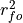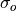# climpred.metrics._msess_murphy¶

climpred.metrics._msess_murphy(forecast, verif, dim=None, **metric_kwargs)[source]

Murphy’s Mean Square Error Skill Score (MSESS).whererepresents the Pearson product-moment correlation coefficient between the forecast and verification data andrepresents the standard deviation of the verification data over the experimental period. See conditional_bias and unconditional_bias for their respective formulations.

Parameters
• forecast (xarray object) – Forecast.

• verif (xarray object) – Verification data.

• dim (str) – Dimension(s) to perform metric over.

• metric_kwargs (dict) – see pearson_r(),

:param mean() and std():

Details:
 minimum -∞ maximum 1.0 perfect 1.0 orientation positive
Reference:

Example

>>> HindcastEnsemble = HindcastEnsemble.remove_bias(alignment='same_verifs')
>>> HindcastEnsemble.verify(metric='msess_murphy', comparison='e2o',
...     alignment='same_verifs', dim='init')
<xarray.Dataset>